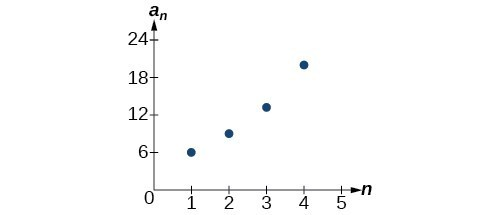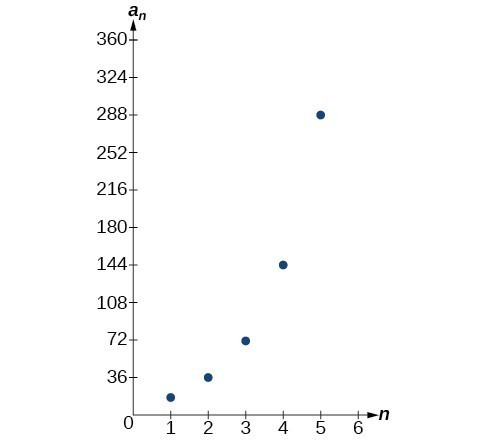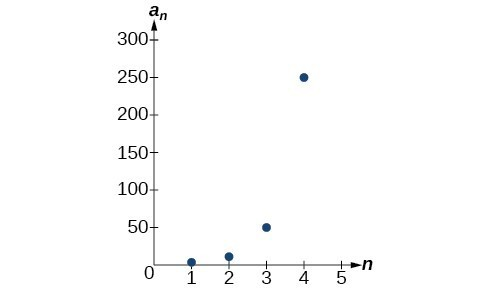## Writing Terms of Geometric Sequences

Now that we can identify a geometric sequence, we will learn how to find the terms of a geometric sequence if we are given the first term and the common ratio. The terms of a geometric sequence can be found by beginning with the first term and multiplying by the common ratio repeatedly. For instance, if the first term of a geometric sequence is ${a}_{1}=-2$ and the common ratio is $r=4$, we can find subsequent terms by multiplying $-2\cdot 4$ to get $-8$ then multiplying the result $-8\cdot 4$ to get $-32$ and so on.

$\begin{array}{l}{a}_{1}=-2\hfill \\ {a}_{2}=\left(-2\cdot 4\right)=-8\hfill \\ {a}_{3}=\left(-8\cdot 4\right)=-32\hfill \\ {a}_{4}=\left(-32\cdot 4\right)-128\hfill \end{array}$

The first four terms are $\left\{-2\text{, }-8\text{, }-32\text{, }-128\right\}$.

### How To: Given the first term and the common factor, find the first four terms of a geometric sequence.

1. Multiply the initial term, ${a}_{1}$, by the common ratio to find the next term, ${a}_{2}$.
2. Repeat the process, using ${a}_{n}={a}_{2}$ to find ${a}_{3}$ and then ${a}_{3}$ to find ${a}_{4,}$ until all four terms have been identified.
3. Write the terms separated by commons within brackets.

### Example 2: Writing the Terms of a Geometric Sequence

List the first four terms of the geometric sequence with ${a}_{1}=5$ and $r=-2$.

### Solution

Multiply ${a}_{1}$ by $-2$ to find ${a}_{2}$. Repeat the process, using ${a}_{2}$ to find ${a}_{3}$, and so on.

$\begin{array}{l}{a}_{1}=5\hfill \\ {a}_{2}=-2{a}_{1}=-10\hfill \\ {a}_{3}=-2{a}_{2}=20\hfill \\ {a}_{4}=-2{a}_{3}=-40\hfill \end{array}$

The first four terms are $\left\{5,-10,20,-40\right\}$.

### Try It 3

List the first five terms of the geometric sequence with ${a}_{1}=18$ and $r=\frac{1}{3}$.

Solution

## Using Recursive Formulas for Geometric Sequences

A recursive formula allows us to find any term of a geometric sequence by using the previous term. Each term is the product of the common ratio and the previous term. For example, suppose the common ratio is 9. Then each term is nine times the previous term. As with any recursive formula, the initial term must be given.

### A General Note: Recursive Formula for a Geometric Sequence

The recursive formula for a geometric sequence with common ratio $r$ and first term ${a}_{1}$ is

${a}_{n}=r{a}_{n - 1},n\ge 2$

### How To: Given the first several terms of a geometric sequence, write its recursive formula.

1. State the initial term.
2. Find the common ratio by dividing any term by the preceding term.
3. Substitute the common ratio into the recursive formula for a geometric sequence.

### Example 3: Using Recursive Formulas for Geometric Sequences

Write a recursive formula for the following geometric sequence.

$\left\{6\text{, }9\text{, }13.5\text{, }20.25\text{, }…\right\}$

### Solution

The first term is given as 6. The common ratio can be found by dividing the second term by the first term.

$r=\frac{9}{6}=1.5$

Substitute the common ratio into the recursive formula for geometric sequences and define ${a}_{1}$.

$\begin{array}{l}{a}_{n}=r{a}_{n - 1}\\ {a}_{n}=1.5{a}_{n - 1}\text{ for }n\ge 2\\ {a}_{1}=6\end{array}$

### Analysis of the Solution

The sequence of data points follows an exponential pattern. The common ratio is also the base of an exponential function as shown in Figure 2.Figure 2

### Do we have to divide the second term by the first term to find the common ratio?

No. We can divide any term in the sequence by the previous term. It is, however, most common to divide the second term by the first term because it is often the easiest method of finding the common ratio.

### Try It 4

Write a recursive formula for the following geometric sequence.

$\left\{2\text{, }\frac{4}{3}\text{, }\frac{8}{9}\text{, }\frac{16}{27}\text{, }…\right\}$

Solution

## Using Explicit Formulas for Geometric Sequences

Because a geometric sequence is an exponential function whose domain is the set of positive integers, and the common ratio is the base of the function, we can write explicit formulas that allow us to find particular terms.

${a}_{n}={a}_{1}{r}^{n - 1}$

Let’s take a look at the sequence $\left\{18\text{, }36\text{, }72\text{, }144\text{, }288\text{, }…\right\}$. This is a geometric sequence with a common ratio of 2 and an exponential function with a base of 2. An explicit formula for this sequence is

${a}_{n}=18\cdot {2}^{n - 1}$

The graph of the sequence is shown in Figure 3.Figure 3

### A General Note: Explicit Formula for a Geometric Sequence

The nth term of a geometric sequence is given by the explicit formula:

${a}_{n}={a}_{1}{r}^{n - 1}$

### Example 4: Writing Terms of Geometric Sequences Using the Explicit Formula

Given a geometric sequence with ${a}_{1}=3$ and ${a}_{4}=24$, find ${a}_{2}$.

### Solution

The sequence can be written in terms of the initial term and the common ratio $r$.

$3,3r,3{r}^{2},3{r}^{3},..$.

Find the common ratio using the given fourth term.

$\begin{array}{ll}{a}_{n}={a}_{1}{r}^{n - 1}\hfill & \hfill \\ {a}_{4}=3{r}^{3}\hfill & \text{Write the fourth term of sequence in terms of }{\alpha }_{1}\text{and }r\hfill \\ 24=3{r}^{3}\hfill & \text{Substitute }24\text{ for}{a}_{4}\hfill \\ 8={r}^{3}\hfill & \text{Divide}\hfill \\ r=2\hfill & \text{Solve for the common ratio}\hfill \end{array}$

Find the second term by multiplying the first term by the common ratio.

$\begin{array}{ll}{a}_{2}\hfill & =2{a}_{1}\hfill \\ \hfill & =2\left(3\right)\hfill \\ \hfill & =6\hfill \end{array}$

### Analysis of the Solution

The common ratio is multiplied by the first term once to find the second term, twice to find the third term, three times to find the fourth term, and so on. The tenth term could be found by multiplying the first term by the common ratio nine times or by multiplying by the common ratio raised to the ninth power.

### Try It 5

Given a geometric sequence with ${a}_{2}=4$ and ${a}_{3}=32$ , find ${a}_{6}$.

Solution

### Example 6: Writing an Explicit Formula for the nth Term of a Geometric Sequence

Write an explicit formula for the $n\text{th}$ term of the following geometric sequence.

$\left\{2\text{, }10\text{, }50\text{, }250\text{, }…\right\}$

### Solution

The first term is 2. The common ratio can be found by dividing the second term by the first term.

$\frac{10}{2}=5$

The common ratio is 5. Substitute the common ratio and the first term of the sequence into the formula.

$\begin{array}{l}{a}_{n}={a}_{1}{r}^{\left(n - 1\right)}\hfill \\ {a}_{n}=2\cdot {5}^{n - 1}\hfill \end{array}$

The graph of this sequence in Figure 4 shows an exponential pattern.Figure 4

### Try It 6

Write an explicit formula for the following geometric sequence.

$\left\{-1\text{, }3\text{, }-9\text{, }27\text{, }…\right\}$